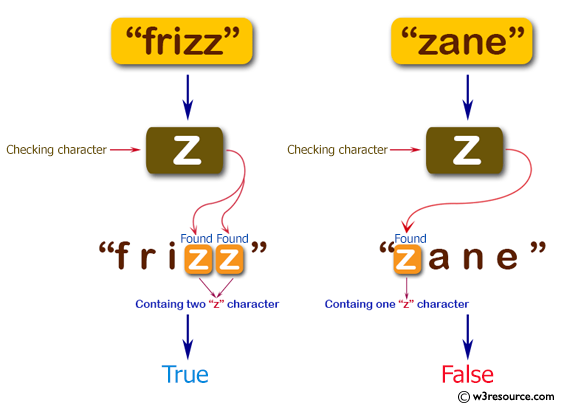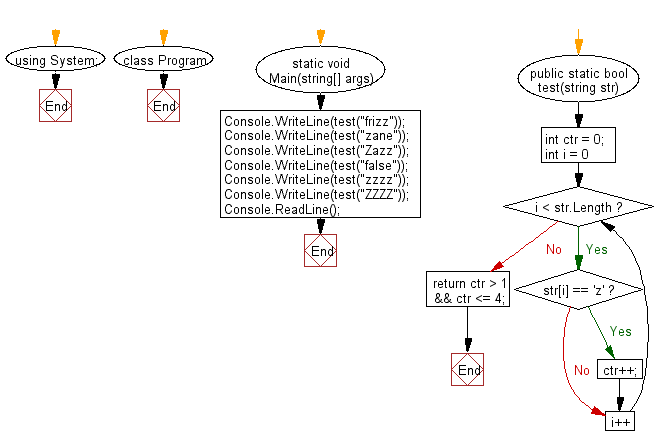﻿ C# - Check a string contains the character z, 2 to 4 times# C# Sharp Basic Algorithm Exercises: Check whether a given string contain the character 'z' from 2 to 4 times

## C# Sharp Basic Algorithm: Exercise-22 with Solution

Write a C# Sharp program to check whether a given string contain the character 'z' from 2 to 4 times.

Pictorial Presentation:Sample Solution:

C# Sharp Code:

``````using System;
namespace exercises
{
class Program
{
static void Main(string[] args)
{
Console.WriteLine(test("frizz"));
Console.WriteLine(test("zane"));
Console.WriteLine(test("Zazz"));
Console.WriteLine(test("false"));
Console.WriteLine(test("zzzz"));
Console.WriteLine(test("ZZZZ"));
}
public static bool test(string str)
{
int ctr = 0;
for (int i = 0; i < str.Length; i++)
{
if (str[i] == 'z')
{
ctr++;
}
}
return ctr > 1 && ctr <= 4;
}
}
}
```
```

Sample Output:

```True
False
True
False
True
False
```

Flowchart:C# Sharp Code Editor:

Improve this sample solution and post your code through Disqus

What is the difficulty level of this exercise?

Test your Programming skills with w3resource's quiz.

﻿